Calculations can be added in to a worksheet. These can either be shown or hidden from the worksheet view.

Calculations can utilise a free type value or can pull from a result from another field defined in the worksheet.

N.B. If using fields from the worksheet they must be either a Number or Select List - Single field.

Running Total - a sum of all of the fields that are toggled on.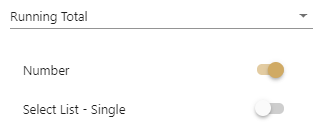Percentage - calculating the percentage of one field/value against another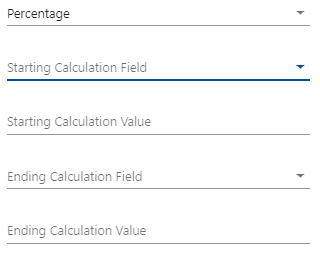Average - Summing the selections and dividing by the number of fields selected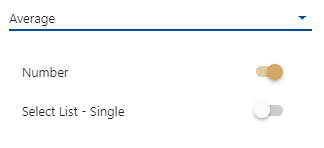Custom - Here you can select a starting and ending field or value and then choose to + - / * %. Fields that can be selected for a custom calculation are either numbers or Single Select Lists that have a value allocated to the selection.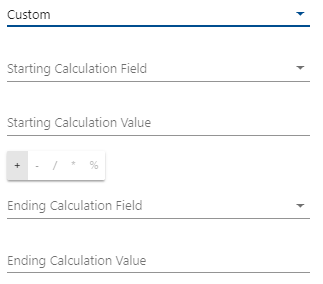Calculations can be built up with in the system by creating calculations of calculations. In this case you may prefer to hide calculation from your worksheet. To do this toggle on 'hide calculation'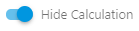Numbers will be displayed in decimals unless 'whole number' is selected.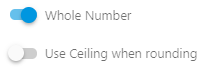When whole number is selected rounding will automatically be to the nearest whole number. If you require for the number to be rounded up select 'use ceiling when rounding'.